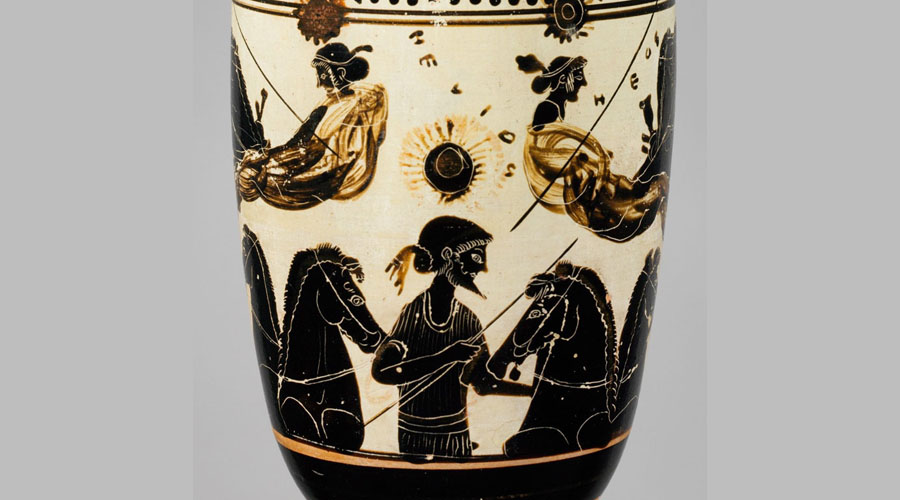# The Archimedes Cattle Problem (KEITH DEVLIN)## 07 Aug The Archimedes Cattle Problem (KEITH DEVLIN)

In the third century BC, the famous Greek mathematician Archimedes issued a challenge to the Alexandrian mathematicians, headed by Eratosthenes. Written in the form of an epigram, Archimedes’s challenge begins thus:

“Compute, o friend, the number of oxen of the Sun, giving thy mind thereto, if thou has a share of wisdom.”

He then goes on to describe, in wonderfully poetic language, a certain herd of cattle, consisting of four types, with bulls and cows of each type. The number of cattle in each of the eight categories is not given, but these numbers are related by nine simple conditions which Archimedes spells out. For example, one of these conditions is that the number of white bulls is equal to the number of yellow bulls plus five-sixths of the number of black bulls. The problem is to determine the number of cattle of each category, and thence the size of the herd. (Actually, what is required is the smallest possible number, since the nine conditions do not imply a unique answer. I’ll give the actual problem in a moment.)

In his epigram, Archimedes goes on to say that anyone who solves the problem would be “not unknowing nor unskilled in numbers, but still not yet to be numbered among the wise.” Nothing could be more apt, since there was to elapse 2,000 years before a computer finally found the solution. Clearly Archimedes had a mischievous streak in addition to his principles, and was trying to pull a fast one on his Alexandrian rivals.

In 1880, a German mathematician called Amthor showed that the total number of cattle in Archimedes herd had to be a number with 206,545 digits, beginning with 7766. Not surprisingly, Amthor gave up at that point. Over the next 85 years, a further 40 digits were worked out. But it was not until 1965 when mathematicians at the University of Waterloo in Canada finally found the complete solution. It took over seven and a half hours of computation on an IBM 7040 computer. Unfortunately, no one thought to keep the printout of the answer! The world had to wait until the problem was solved a second time using a Cray-1 computer in 1981 for a published printout. It took the Cray just 10 minutes to crack it. But after 2,000 years I think Archimedes has to have the last laugh.

So what is Archimedes’ Cattle Problem?

Archimedes asks you to imagine a certain herd of cattle consisting of both cows and bulls, each of which may be white, black, yellow, or dappled. The numbers of each category of cattle are connected by various, simple conditions. To give these, let W denote the number of white bulls, w the number of white cows, B the number of black bulls, b the number of black cows, with Y, y and D, d playing analogous roles for the other colors. Using Archimedes’ method of writing fractions (that is, utilizing only simple reciprocals), the fist seven conditions which these various numbers have to satisfy are:

(1) W = (1/2 + 1/3)B + y
(2) B = (1/4 + 1/5)D + Y

(3) D = (1/6 + 1/7)W + Y

(4) w = (1/3 + 1/4)(B + b)

(5) b = (1/4 + 1/5)(D + d)

(6) d = (1/5 + 1/6)(Y + y)

(7) y = (1/6 + 1/7)(W + w)

The two remaining conditions are:
(8) W + B is a perfect square
(9) Y + D is a triangular number.

[A triangular number is one that is equal to a number of balls that may be arranged to form a triangle, which is the same as saying that the number must be of the form n(n+1)/2 for some n.]
The problem is to determine the size of the eight unknowns, and thus the size of the herd. More precisely, the aim is to find the least solution, since the conditions admit more than one solution. If conditions (8) and (9) are dropped, the problem is relatively easy. The smallest herd consists of 50,389,082 cattle. The additional two conditions make the problem considerably harder. It has been claimed that the first complete solution was worked out by the Hillsboro (Illinois) Mathematics Club between 1889 and 1893, although no copy of their solution has ever been found, and there is some evidence to suggest that what they in fact did was work out some of the digits of the 206,545 digit solution and provide an algorithm for the computation of the remainder.

In 1965, H. C. Williams, R. A. German, and C. R. Zarnke at the University of Waterloo in Canada used an IBM 7040 computer to crack the problem once and for all. The final solution occupied 42 sheets of print-out. In 1981, Harry Nelson repeated the calculation using a Cray-1. This machine took a mere 10 minutes to come up with the answer. Reduced to fit 12 pages of print-out on a single journal page, the solution was published in the Journal of Recreational Mathematics 13 (1981), pp.162-176.

Mathematician Keith Devlin
The Millennium Problems: The Seven Greatest Unsolved Mathematical Puzzles of Our Time, published by Basic Books (hardback 2002, paperback 2003).